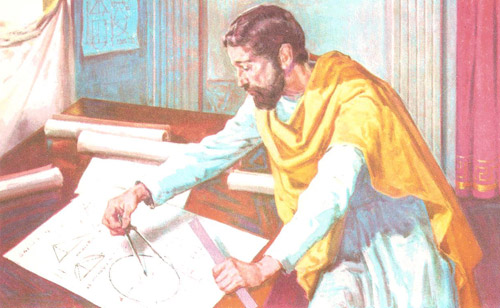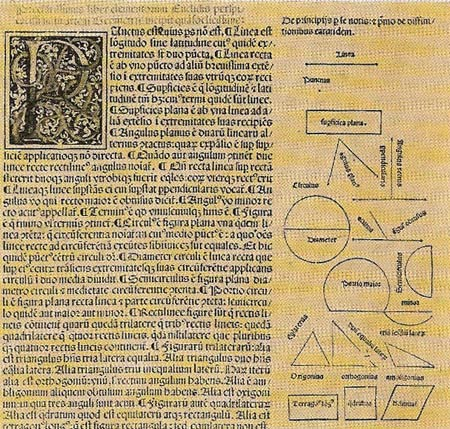A# Euclid of Alexandria (c. 330–c. 270 BC)Figure 1. Artist's impression of Euclid drawing geometrical figures in his study.Figure 2. The great basic text on Greek geometry was Euclid's Elements

Euclid was a Greek mathematician who compiled and systematically arranged the geometry and number theory of his day into the famous text Elements (see Figure 2). This text, used in schools for about 2,000 years, earned him the name "the father of geometry." Even today, the geometries which don't satisfy the fifth of Euclid's "common notions" (now called axioms or postulates) are called non-Euclidean geometries.

Little is known of Euclid's life. Proclus wrote (c. AD 350) that Euclid lived during the reign of Ptolemy and founded the first school of mathematics in Alexandria – the site of the most impressive library of ancient times with perhaps as many as 700,000 volumes. He wrote books on other subjects such as optics and conic sections, but most of them are now lost.

## Euclidean geometry

Geometry means literally 'measurement of the earth' – what we should now call 'surveying'. It arose out of the practical needs of the agricultural peoples of ancient Mesopotamia (modern Iraq) and Egypt, for they had to measure out and apportion plots of land. In Egypt, the yearly flooding of the Nile, which fertilized the land as it still does today, meant that the boundaries of the fields had to be worked out again, after being washed away.

These primitive ways of measuring spaces came next to be applied to building. The ancient temples of Egypt and Mesopotamia, their roads and canals, all bear witness to the geometrical skills their makers had attained. Records survive which show how far they had gone. Nevertheless, none of this is really 'geometry' as we know it. The word was coined by the Greeks, because it is they who first turned all the practical rules of measurement into an ordered branch of mathematics.

To later generations, Greek mathematics came to mean Euclid. Yet for nearly three centuries before his time there had been Greek mathematicians. The earliest mathematical proofs are attributed to Thales of Miletus, who lived about 585 BC, whereas we know that Euclid was at work in 300 BC. He taught at Alexandria, which lies at the western end of the Nile delta. The city had been enlarged and given its new name by Alexander the Great in 332 BC, and was fast becoming the greatest center of Greek influence outside Greece: what we now call 'Hellenistic culture'.

It seems that Euclid was not so much an inventor as a recorder of the findings of others: as such he was the greatest mathematical schoolmaster of all time. His Elements is the only textbook that has ever been used for well over 2,000 years. Not until the nineteenth century did mathematicians show more precision than Euclid. Until then the Elements had been regarded as the most perfect example of a theory in which conclusions are drawn from assumptions in a systematic way: what we call a 'deductive theory'. From a set of five basic assumptions or axioms (including the controversial parallel postulate), with careful definitions and logical reasoning, he derived a vast array of theorems set out in an orderly way.

Euclidean geometry is commonly split up into plane geometry, which is concerned with figures and constructions in two or less dimensions (such as the polygon, circle, ellipse, curve, line, and point), and solid or three-dimensional geometry, which deals with three-dimensional figures (such as the polyhedron, sphere, and ellipsoid).

## Euclidean space

Euclidean space is any n-dimensional mathematical space that is a generalization of the familiar two- and three-dimensional spaces described by the axioms of Euclidean geometry. The term "n-dimensional Euclidean space" (where n is any positive whole number) is usually abbreviated to "Euclidean n-space", or even just "n-space". Formally, Euclidean n-space is the set Rn (where R is the set of real numbers) together with the distance function, which is obtained by defining the distance between two points (x1, ..., xn) and (y1, ...,yn) to be the square root of (sigma) (xi - yi)2, where the sum is over i = 1, ..., n. This distance function is based on Pythagoras' theorem and is called the Euclidean metric.

## Non-Euclidean geometry

Main article.

To Euclid, the axioms were obvious: in no need of explanation. Today, we no longer feel bound to be as sure as he was. For instance, he took it that if two straight lines cutting a third interior angles (angles on the same side) amounting to less than two right angles, then those two lines will eventually meet. This means that through any point outside a line there is one, and only one, line that will not meet the first: what is called a line 'parallel' to it. This for Euclid was an axiom. We no longer hold this to be obvious. For 200 years now mathematics have tried to derive the parallel axiom from other assumptions of Euclidean geometry, but in vain. If we simply drop the parallel axiom, we can build up other systems of geometrical theorems which hang together just as well. Such systems are called 'non-Euclidean', and they have become very important in modern science. Einstein's general theory of relativity makes use of one of these non-Euclidian geometries.

As far back as the sixth century BC Pythagoras had discovered that the diagonal of a square could not be measured as an ordinary proportion of the side of a square. He called the diagonal 'unmeasurable', which the Romans translated into 'irrational', the word we use today. These irrational numbers were a great blow to the mathematicians of that time, because they had wrongly thought that all lengths could be measured as proportions that could be stated in whole numbers. However, they worked out a method of using whole numbers to get closer and closer to these measurements. But on the whole their methods for finding proportions were geometrical: Euclid's 'Elements' deal with them at length. They also deal with arithmetic, and provide proofs for some of the basic elementary theorems about numbers. Especially famous is a proof about prime numbers (those numbers that have no factors): Euclid showed that, however big a prime number might be, there are always still bigger ones.

Euclid also wrote on other subjects besides mathematics. Many of his books are lost, but his texts on optics and music have survived.

 Two anecdotes about Euclid have come down to us. They show what kind of man and teacher he was. When, according to the Greek philosopher Proclus, the Egyptian ruler Ptolemy asked if there was a shorter way to the study of geometry than the Elements, Euclid replied: "There is no royal road to geometry." Euclid once heard one of his students ask what gain would come from studying geometry. The teacher promptly called a slave and told him to give the pupil a small coin, "since he must needs make gain from all he learns."